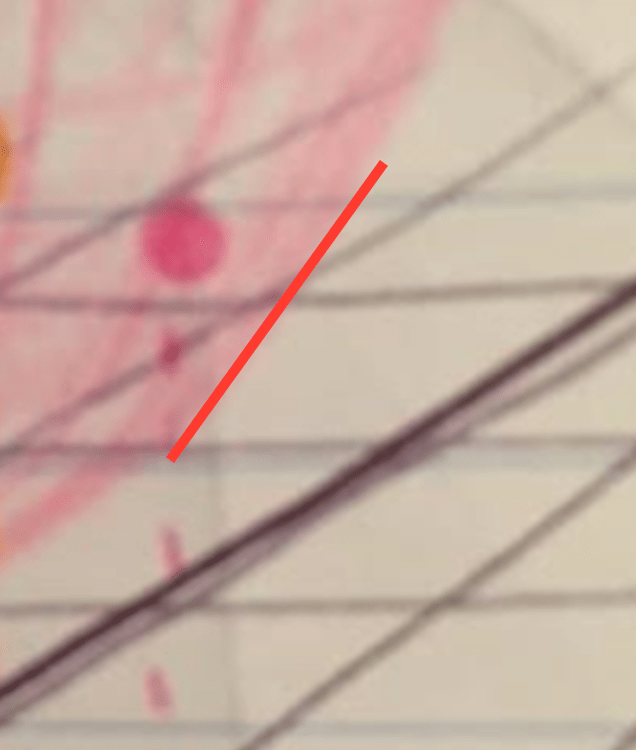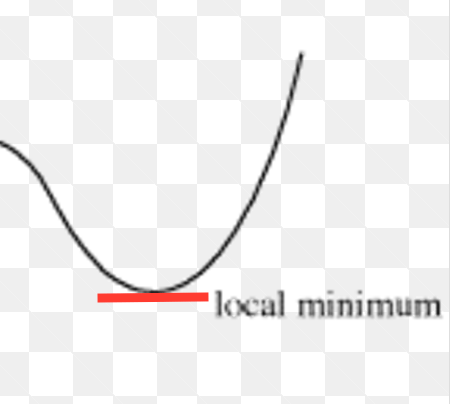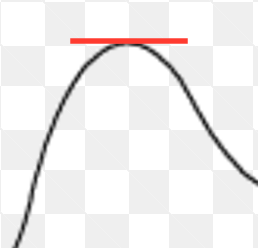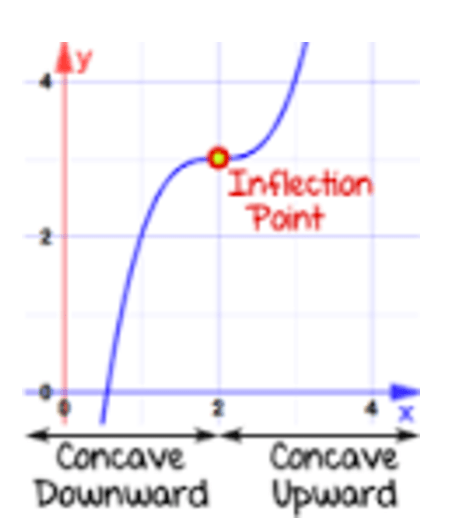# Machine Learning Intuition: Using Derivatives to Minimize the Cost Function

We have talked before about the intuition behind cost function optimization in machine learning. We took a look at where cost functions come from and what they look like. We also talked about how one might get to the bottom of one with gradient descent.

When you open a machine learning textbook, you’ll see much more math than we used in that introduction. But the math is, in fact, important; the math gives us tools that we can use to quickly find the minima of our cost functions.

We’re going to talk about two of those tools: derivatives (in this post) and Taylor series (in the next post). Both of these tools come from calculus and help us identify where to find the minima on our cost functions.

Imagine you have a cost function that looks like this:You want to find the m and b values for which the error is the lowest. That is, you want to find the bottom of this cost function.

We discussed how to do this by plotting points and using gradient descent. This is a useful, generalizable, and omnipresent approach in machine learning.

This cost function in particular, though, provides us with a few advantages that give us a way to find its minimum with a few calculations.

1. Our cost function is differentiable everywhere.

Differentiable? What is that? Differentiable means that, at any point on this function, we can approximate what this function looks like with a much simpler function that describes how fast this function is changing.So if we zoom in on just one little part of the function like this:We can say “Well, this function is not flat, but for this little area we can use a function that is flat to approximate where the errors are.”

Like so:Our approximation is not a fancy parabola…it’s just a line. But for that little area of the function, it pretty closely approximates what the real function is doing. It’s describing the rate of change of the function…the slope at that point on the function. A parabola is flat at the bottom and gets steeper and steeper as you move away from the bottom. The derivative describes for us the function’s slope. The slope of a flat function is zero. So when the derivative is zero, we know that this is where the function is flat. As the slope gets bigger, we know that the function is steeper here.

Did that last paragraph give you a clue as to why the derivative matters? When the derivative (the slope of the function we’re deriving) is zero, that tells us that the function is flat here. Parabolas are flat…at the bottom.

By using the derivative to figure out where the function is flat, we can find the bottom!

Not all cost functions are parabolas. But we can use the derivative of any differentiable function, from the simple parabola to the wavy f(x) = sin(x) function, to figure out where that function is flat. For the function to change direction and give us a local minimum, there has to be a flat part:How do you find the derivative?

Depends on the function: we know lots of cool stuff about derivatives, so if you’re super interested in derivatives I recommend checking out Paul’s Math Notes on them. It’s a brief document that catalogs the most important things about derivatives without really explaining them. But you can look up the things on there that you don’t understand until you know everything about derivatives that you’ll ever need for machine learning, and then some. Looking things up is the most important skill you can develop as a tech person.

That having been said, this is the part of Paul’s Math Notes that we need to find the derivative of a parabola:The equation for parabolas follow the pattern f(x)ax2 + bx + c.  So to get the derivative of this function, f'(x), we multiply each power by its coefficient, and then reduce the power.

f(x)ax2 + bx + c  = ax2 + bx(1) + c(0)
f'(x) = 2* ax(2-1) + 1*bx(1-1)
(the constant term, c, is removed above because all it does is move a function up and down…it doesn’t tell us anything about the slope of the function. y=2x+1 and y=2x have the same slope, for example).
f'(x) = 2 ax(1) + b*1
(the x in bx goes away above because 1-1 is zero, and anything to the zero power is 1).
f'(x) = 2 ax + b
(the x is just x now because anything to the first power is equal to itself).

So we have our derivative function: f'(x) = 2 ax + b. To find out where a parabola is flat, we have to find out where this function is equal to zero.

For example, let’s say that we have a cost function that describes error relative to the slope of a regression line, and our cost function looks like f(x) =3x2 + 6x + 4. Where is the slope of this parabola equal to zero?

f'(x) = 2 *3x + 6
0 = 2 *3x + 6
0 = 6x + 6
-6 = 6x

x = -1.

The slope is zero at -1. The parabola is flat at -1. So at a slope of -1, we have our minimum cost.

Now, our pink parabola isn’t actually a parabola: it’s a paraboloid, and it has two dimensions: slope and y-intercept, not just slope. So to find the minimum, we would have to find the partial derivatives where the slope of the cost function in the m direction is zero and the slope of the cost function in the b direction is also zero. To do this, we just hold m constant to find the minimum in the b direction and then hold b constant to find the minimum in the m direction. This illustrated example explains it well, but the intuition of it is exactly the same as we saw for gradient descent: pretend one variable is the same all the time and find the minimum on the other one, then pretend the other variable is the same all the time and see if we can’t get an even lower cost that way. Derivatives work the same way regardless of the direction you’re minimizing. This continues to work when we’re minimizing a cost function on many dimensions—say, if we’re fitting a line to housing prices based on the five dimensions of location, size, color, school district, and friendliness of neighbors. (No one takes that last one into account when moving. Giant mistake.)

Now, if you’ve been thinking about where functions are flat, you might have noticed a detail that we left out. Won’t a function’s derivative also be zero….here? Where the cost is locally the highest?Or here? Where the cost does not change direction?

Yes. You are correct! So the derivative is not a foolproof mechanism. And there’s actually another catch, which we already discussed in our introduction to cost function optimization: just because we found a local minimum doesn’t mean we found the minimum for the whole function.

There are ways around these issues: for example, we can use the second derivative, that is, the derivative of the derivative, to figure out if we’re at a local max (when the second derivative is negative) or a local minimum (when the second derivative is positive) or an inflection point (when the second derivative is zero).

But we actually get lucky on a lot of cost functions in machine learning. And that’s where the second advantage of our paraboloid cost function comes in.

2. Our cost function is convex (or, if you prefer, concave up) everywhere.

Let’s look at the second derivative of f(x) =3x2 + 6x + 4.
f'(x) = 6x + 6
f”(x) = 6x(1-1)  =6x(0) = 6

Six is never negative. Six is just six. It’s always positive. So our function is concave up everywhere.

This means that, if we find a spot where the derivative is zero, it has to be a minimum because the function is concave up there. It also means that there is only one minimum, because the function is always concave up, which means it can’t sneakily turn back downward on us anywhere.

And conveniently, many, many cost functions in machine learning have this property. We’ll take a look at some of them in later posts.

When we have a cost function that is differentiable everywhere, we can use the derivative to speed up our process of finding the minima. And when the cost function is also convex everywhere, we can rest assured that there is one global minimum for us to find.

Once we’re comfortable finding derivatives and where they are equal to zero, the cost function optimization process can go pretty fast! So we want to have this tool in our toolbelt for examining our cost functions for our models.

This site uses Akismet to reduce spam. Learn how your comment data is processed.